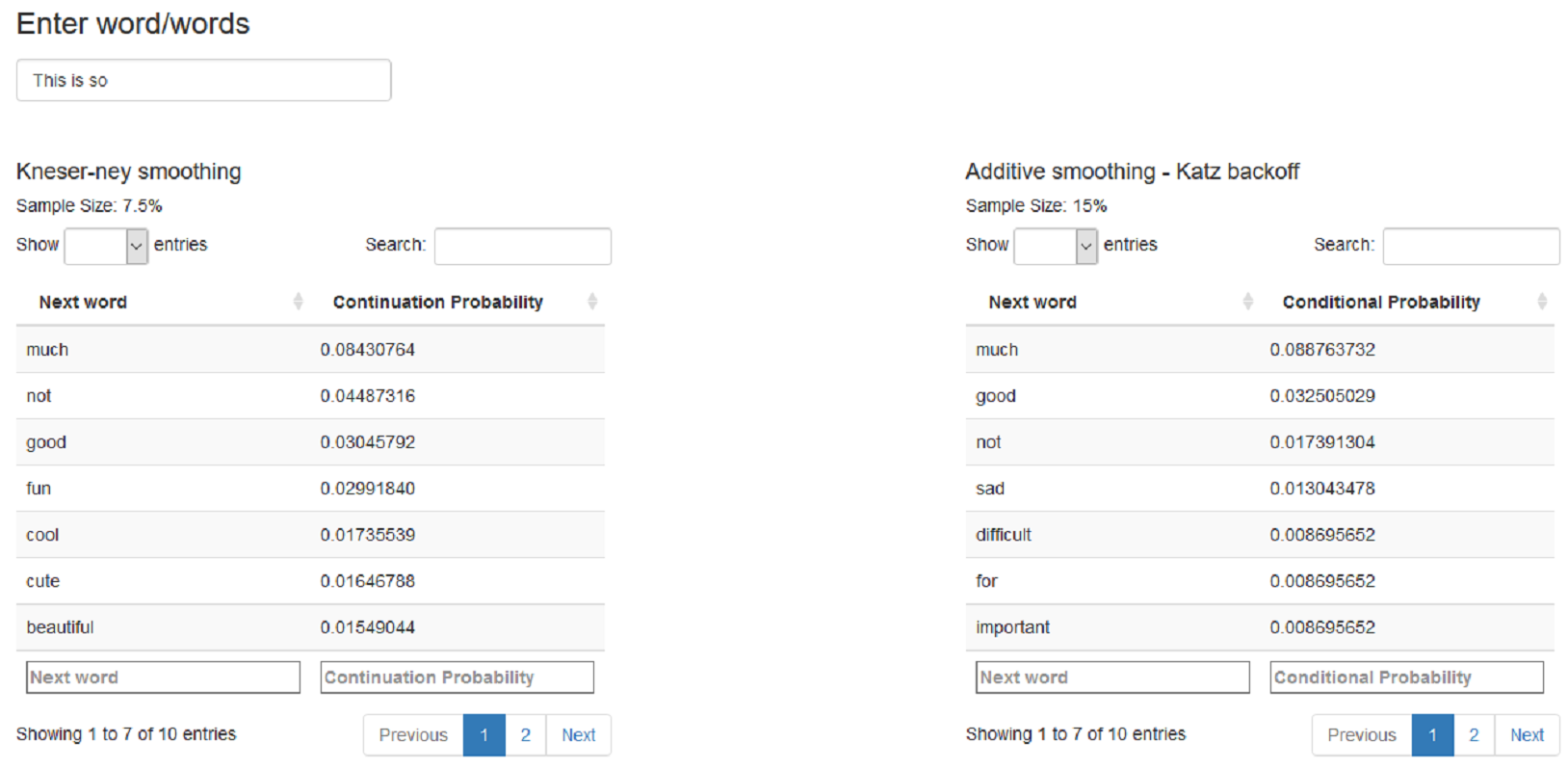# PredictNextWord

Tinniam V Ganesh
22 Aug 2015

### Create and clean the Corpus

This presentation highlights the steps in creating a Word Predict Shiny App

• Ingest the from the Tweets, Blogs and News
• Sample the data train & test data (7.5% for Kneser-Ney smoothing & 10% for Additiive smoothing-Katz backoff)
• Create a Corpus from the tweets, blogs and news items
• Clean the Corpus to remove punctuation, special characters, stopwords etc
• Remove profanity from the training and test set
• Use the package RWeka to create Quadgrams,Trigrams,Bigrams and Unigrams

### Use Laplace Add-1 smoothing & Katz backoff

1. Use Markov chains to calculate the Maximum Likelihood estimate P(C|AB) = count(ABC)/count(AB)
2. For previous terms whose count is 0, perform Laplace Add - 1 smoothing Padd-1(C|AB) = (count(C|AB) + 1)/(count(AB) + V)
3. Use Katz backoff algorithm to back off to lower n-1 grams if not found in n grams
4. Create n-gram csv files with n-1 gram, next word and conditional probability

### Kneser-Ney smoothing

The Kneser-Ney smoothing is based on determining the 'continuation probability' of the next word.

The Kneser-Ney formula is given below $$P_{\mathit{KN}}(w_i \mid w_{i-1}) = \dfrac{\max(c(w_{i-1} w_i) - \delta, 0)}{\sum_{w'} c(w_{i-1} w')} + \lambda \dfrac{\left| \{ w_{i-1} : c(w_{i-1}, w_i) > 0 \} \right|}{\left| \{ w_{j-1} : c(w_{j-1},w_j) > 0\} \right|}$$ where $$\delta$$ is the 'discount' and $$\lambda$$ is a normalizing constant

$$\lambda(w_{i-1}) = \dfrac{\delta}{c(w_{i-1})} \left| \{w' : c(w_{i-1}, w') > 0\} \right|.$$

Create n-grams csv file with n-1 gram, next word and continuation probability

### Text mining and performance tuning

a) Additive smoothing+ Katz backoff b) Kneser-Ney smoothing processed as follows

1. Sample size was chosen iteratively based on space and performance requirements
2. tm and Rweka package was used for cleaning and creation of n-grams
3. dplyr commands and data table were found to improve performance
4. Vectorizing operations using 'sapply' instead of 'for' loops speeded up processing many times over.Thank You!